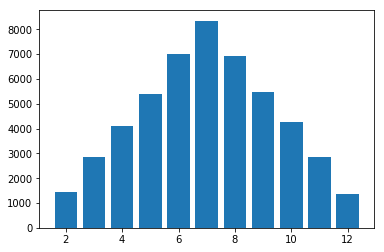### If you roll two dice, how likely is it that your sum is greater than 7?¶

In :
from random import choices
from collections import Counter

In :
# Define a 6-sided die
faces = list(range(1, 7))
faces

Out:
[1, 2, 3, 4, 5, 6]
In :
# A function for rolling a pair of dice
roll_2_dice = (lambda: choices(population=faces, weights=None, k=2))
pair = roll_2_dice()
pair

Out:
[4, 3]
In :
sum(pair)

Out:
7
In :
# Simulate rolling a pair many times and tracking the outcomes
rolls = [sum(roll_2_dice()) for _ in range(50_000)]
#print(rolls)

In :
Counter?

Init signature: Counter(*args, **kwds)
Docstring:
Dict subclass for counting hashable items.  Sometimes called a bag
or multiset.  Elements are stored as dictionary keys and their counts
are stored as dictionary values.

>>> c = Counter('abcdeabcdabcaba')  # count elements from a string

>>> c.most_common(3)                # three most common elements
[('a', 5), ('b', 4), ('c', 3)]
>>> sorted(c)                       # list all unique elements
['a', 'b', 'c', 'd', 'e']
>>> ''.join(sorted(c.elements()))   # list elements with repetitions
'aaaaabbbbcccdde'
>>> sum(c.values())                 # total of all counts
15

>>> c['a']                          # count of letter 'a'
5
>>> for elem in 'shazam':           # update counts from an iterable
...     c[elem] += 1                # by adding 1 to each element's count
>>> c['a']                          # now there are seven 'a'
7
>>> del c['b']                      # remove all 'b'
>>> c['b']                          # now there are zero 'b'
0

>>> d = Counter('simsalabim')       # make another counter
>>> c.update(d)                     # add in the second counter
>>> c['a']                          # now there are nine 'a'
9

>>> c.clear()                       # empty the counter
>>> c
Counter()

Note:  If a count is set to zero or reduced to zero, it will remain
in the counter until the entry is deleted or the counter is cleared:

>>> c = Counter('aaabbc')
>>> c['b'] -= 2                     # reduce the count of 'b' by two
>>> c.most_common()                 # 'b' is still in, but its count is zero
[('a', 3), ('c', 1), ('b', 0)]
Init docstring:
Create a new, empty Counter object.  And if given, count elements
from an input iterable.  Or, initialize the count from another mapping
of elements to their counts.

>>> c = Counter()                           # a new, empty counter
>>> c = Counter('gallahad')                 # a new counter from an iterable
>>> c = Counter({'a': 4, 'b': 2})           # a new counter from a mapping
>>> c = Counter(a=4, b=2)                   # a new counter from keyword args
File:           ~/anaconda3/lib/python3.7/collections/__init__.py
Type:           type
Subclasses:

In :
# Count those outcomes (number of sums of paired elements)
rolls_counts = Counter(rolls)
print(rolls_counts)

Counter({7: 8347, 6: 6990, 8: 6912, 9: 5463, 5: 5409, 10: 4282, 4: 4113, 3: 2857, 11: 2841, 2: 1439, 12: 1347})

In :
# Sort by faces  or counts  and store the sorted results as a dictionary
rolls_counts = dict(sorted(rolls_counts.items(), key=lambda x: x, reverse=True))
print(rolls_counts)

{12: 1347, 11: 2841, 10: 4282, 9: 5463, 8: 6912, 7: 8347, 6: 6990, 5: 5409, 4: 4113, 3: 2857, 2: 1439}

In :
# Plot the outcome of simulating many dice rolling (Binomial Distribution)
import matplotlib.pyplot as plt
%matplotlib inline

labels, values = zip(*rolls_counts.items()) # Unpack dict
plt.bar(x=labels, height=values);In :
# If you roll two dice, how likely is it that your sum is greater than 7
sum(v for k, v in rolls_counts.items() if k > 7) / sum(rolls_counts.values())

Out:
0.4169
In :
def duration(seconds):
'''
Encodes seconds into a formated string d:h:m:s.
'''
time = seconds
day = time // (24 * 3600)
time = time % (24 * 3600)
hour = time // 3600
time %= 3600
minutes = time // 60
time %= 60
seconds = time
print("d:h:m:s-> %d:%d:%d:%d" % (day, hour, minutes, seconds))

In :
# If you roll seven dice, how likely is it that your sum is greater than 35?

import time
start = time.time()

faces = list(range(1, 7))
roll_7_dice = (lambda: choices(population=faces, weights=None, k=7))
rolls7 = [sum(roll_7_dice()) for _ in range(400_000_000)]
rolls_counts7 = Counter(rolls7)

likelihood = sum(v for k, v in rolls_counts7.items() if k > 35) / sum(rolls_counts7.values())
print("The likelihood is {}".format(likelihood))

duration(time.time() - start)

The likelihood is 0.00610669
d:h:m:s-> 0:0:13:25

In [ ]:


In [ ]:


In [ ]:


In [ ]:
from random import choices
from collections import Counter

# 6-sided die
faces = list(range(1, 7))

# A function for rolling a pair of dice
roll_2_dice = (lambda: choices(population=faces, weights=None, k=2))

# Simulate rolling a pair many times and tracking the outcomes
rolls = [sum(roll_2_dice()) for _ in range(50_000)]

# Count those outcomes
rolls_counts = Counter(rolls)

# If you roll two dice, how likely is it that your sum is greater than 7
sum(v for k, v in rolls_counts.items() if k > 7) / sum(rolls_counts.values())

In [ ]:


In [ ]:


In [ ]:


In [ ]: